Smartick is a fun way to learn math!Jan19

# Learn about the Importance of the Parentheses in Powers

In this entry, we will learn the importance of the parentheses when performing calculations with signs (negative) in the powers.

We all know that multiplication is an abbreviated form of writing a reiterated sum. For example, 2 + 2 + 2 + 2 + 2 can be more simply written as 2 x 5.

In the same way, we use the powers to express the multiplication of a number by itself several times in a more abbreviated way. For example, 2 x 2 x 2 can be expressed as 2³.

First of all, let’s quickly review the elements of a power.

## Elements of a power

The powers are formed by the base and by the exponent as you see in the following image. The base is the number that is being multiplied several times. The exponent is the number of times the base is being multiplied.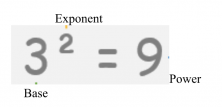After this slight review of the elements of a power, let’s get to the point. The parentheses in the negative base powers are very important. We must keep them in mind when we approach operations. Often they are causes of errors that lead us to erroneous solutions.

### Let’s look at the difference between (-5) ² and -5²

If the base is in parentheses, as in our first case, the exponent affects everything that is inside the parenthesis, that is, the sign and the number.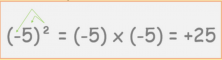However, if the base is not in parentheses, as in the second case, the exponent affects only the immediate value to the left, that is, only the number, without the sign.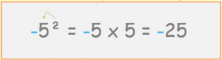As you can see, a pair of parentheses can totally change the result.

Let’s see some more examples of handling parentheses in the powers.

###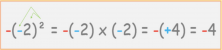Powers: Example 2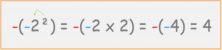It may seem confusing and it takes a bit of practice to get used to the calculations but it is not difficult, so I encourage you to start Smartick and practice this subject among many more subjects in primary school math.

I hope this has helped you. And do not forget the importance of parentheses!

Fun is our brain’s favorite way of learning
Diane Ackerman
Smartick is a fun way to learn math
• 15 fun minutes a day
• Millions of students since 2009• Zvjezdana JovanovicJun 04 2021, 3:20 PM

Great